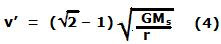## Monday, November 10, 2008

### Irodov Problem 1.233Let us first understand whats happening. When the object is shot out of Earth, if the object's velocity is greater than that of the escape velocity of Earth, it will escape the gravitational field of Earth. However, it may not escape the gravitational field of Sun which is far more massive than the Earth and holds Earth itself within its gravitational force field. The object needs much more velocity to escape the sun itself.

By the time the object escapes Earth's gravitational it would have lost a lot of its kinetic energy and so must have slowed down. Even after slowing down it must possess enough kinetic energy to escape the Sun. To make best use of the Earth's velocity as it revolves around the sun, it is best if the object is imparted a velocity in the direction of Earth's motion as shown in the Figure.

The typical paths of the object and the Earth are depicted in the figure. The object initially shares Earth's velocity and shoots tangentially to Earth's orbit around the sun. Earth's gravitational force and the Sun's gravitational forces will curve the path of the object as it moves as shown in the Figure. For an exact solution we must solve the problem considering these curved paths. This exact solution is non-trivial.

However, considering that the Earth's orbit is very large, we can neglect these exact effects due to the curvature of the Earth's orbit and come up with an approximate solution. Suppose that
the object is shot with a velocity v3 relative to the Earth. Let the mass of Earth be ME and let its radius be RE. Let its mass be m. Let its velocity relative to Earth by the time it escapes Earth's gravitational field be v' relative to Earth. v' can be computed using conservation of energy as,Now the velocity of the object after it has escaped Earth, as seen from a frame fixed on the Sun will be v' + V1 , where V1 is the tangential velocity of the Earth with respect to the Sun. Now let us assume that even after the object has escaped Earth, its distance from Earth is negligible compared to the radius of Earth's orbit (since Earth's orbit around the sun is very very large). In other words after escaping from Earth, the object is at distance of approximately r - the distance of Earth from the Sun.

This, velocity v' + V1 must be sufficient for the object to escape the Sun's gravitational field. In order for the object to escape the Sun's gravity, its total energy (potential + kinetic) must be greater than or equal to 0. At the minimum velocity needed to escape the Sun, its total energy must be 0. So we have,Now, the orbital velocity of Earth around the sun can be obtained by equating its centrifugal force (as seen in the Earth's frame of reference) to the gravitational force from the sun. So we have,From (2) and (3) we have,From (1) and (4) we have,